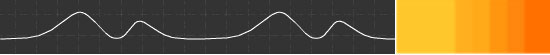## NIPS 2004 - Fast N-Body Learning

Nando de Freitas and Dan Huttenlocher

Many algorithms for statistical inference have a O(N^2) time dependence on the size of the state space (N). For example, at the heart of the belief propagation algorithm is an expensive operation called message passing. The cost of this operation is O(nN^2), where n is the number of nodes in the probabilistic graphical model and N is the number of discrete values the node (random variable) takes. The N^2 component of the cost is particularly large when we discretize a continuous state space using deterministic or Monte Carlo grids.

The N^2 cost arises whenever one computes a kernel density estimate. In addition to message passing, many other machine learning algorithms are subject to this expensive operation. The list includes Gaussian processes, radial basis networks, SVMs, particle smoothing, population Monte Carlo methods, multidimensional scaling and kernel methods for dimensionality reduction, classification, regression and semi-supervised learning. This cost also arises when one is interested in the max operator (as in max belief propagation) and nearest neighbour search.

In recent years, researchers in different communities (physics, numerical computation, machine learning and computer vision) have put forward algorithms to reduce this cost to O(NlogN) and even O(N) in some cases. Examples of these techniques include fast multipole methods, the fast Gauss transform, distance transforms, kd-trees, ball trees, dual tree recursions and several other more specialized techniques. The workshop will bring many of these researchers together.

The target audience is anyone who would like to see their favourite machine learning algorithm running in seconds as opposed to hours or days.